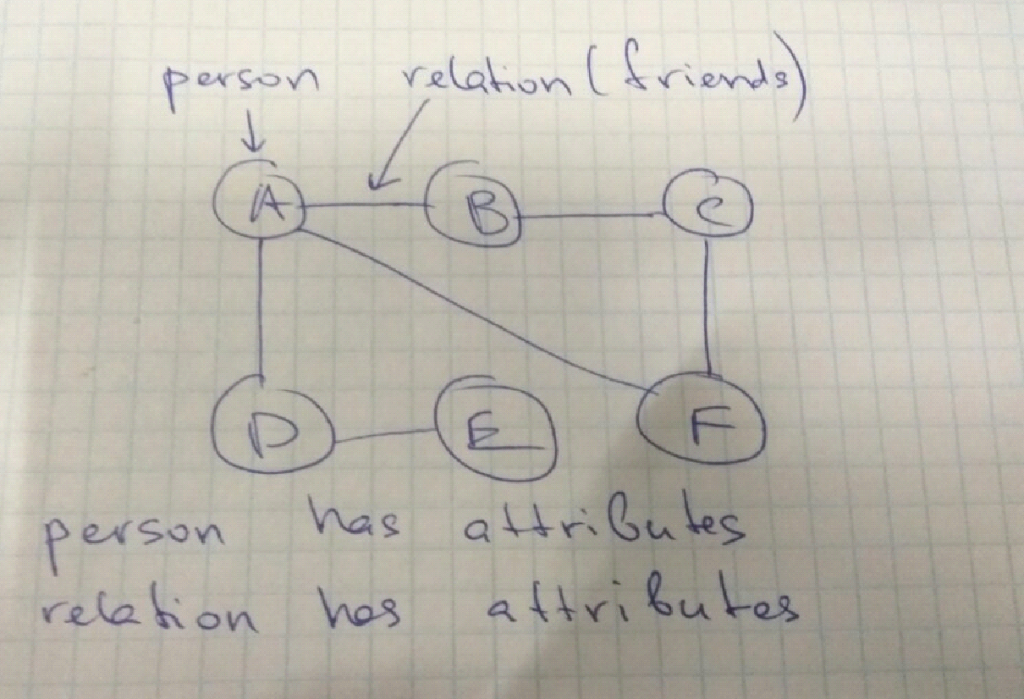# Python Graph Network Example

## Graph Network for a Set of People Using Python

The idea is to build a network of people using graph methodology. In the graph, network nodes will represent people and edges will represent some kind of connection between them.

Every person has its name and attributes. Every relation has its name and attributes.

I represented the idea on the paper:It is an interesting task, because in fact all social networks use a similar model to connect people.

Here is the source code in Python with comments:

```class Network(object):
def __init__(self):
self.__network_dict = {}
self.__person_property = {}
self.__relation_property = {}

def create_person(self):
print("enter person")
person = input()
if person not in self.__network_dict:
self.__network_dict[person] = []
#print(self.__network_dict)

if person in self.__network_dict:
if person not in self.__person_property:
self.__person_property[person] = {prop: value}
else:
self.__person_property[person].update({prop: value})

if person1 and person2 in self.__network_dict:
self.__network_dict[person1].append(person2)
self.__network_dict[person2].append(person1)
else:
self.__network_dict[person1] = [person2]
self.__network_dict[person2] = [person1]

def add_relation_property(self, person1, person2, prop, value):
prop_set = frozenset([person1, person2])
if person1 in self.__network_dict and person2 in self.__network_dict[person1]:
if prop_set not in self.__relation_property:
self.__relation_property[prop_set] = {prop: value}
else:
self.__relation_property[prop_set].update({prop: value})
#print(self.__relation_property)

def get_person(self, name):
if name in self.__network_dict:
return (self.__network_dict[name])

def friends_of_friends(self, name):
# get a list of friends of friends of the person with given name
friend_of_friends = []
if name in self.__network_dict:
for friend in self.__network_dict[name]:
friend_of_friends.append(friend)
for person in self.__network_dict[friend]:
friend_of_friends.append(person)
#print(self.__network_dict)
return list(set(friend_of_friends))

if __name__ == "__main__":
"""EXAMPLE"""
network = Network()
for i in range(2):
network.create_person()
for i in range(2):
print("enter person1")
person1 = input()
print("enter person2")
person2 = input()
print("enter person's name")
print("enter person name")
person1 = input()
person2 = input()
print("prop")
prop = input()
print("value")
value = input()

print("enter person's name")
print("enter person name")
person1 = input()
person2 = input()
print("prop")
prop = input()
print("value")
value = input()
```

That’s how we can create a simplified model of a social network. We can easily create users and connections between them, and add properties to both users and relations.